## Find the area of equilateral triangle with side a. Please answer with completely simplified exact values. Answer: A = sq. Units

Question

Find the area of equilateral triangle with side a. Please answer with completely simplified exact values. Answer: A = sq. Units

in progress 0
2 weeks 2021-09-02T20:38:43+00:00 1 Answers 0 views 0

1. Solution :

It is given that the length of a side of a triangle is given by ‘a’.

It is an equilateral triangle.

So the three sides will be of equal length and is a, a, a units.

Now the semi perimeter of the equilateral triangle is given by :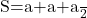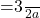Therefore, using the Heron’s formula, we can find the area of the equilateral triangle.

Area of the equilateral triangle is given by :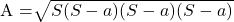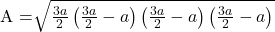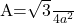square units.